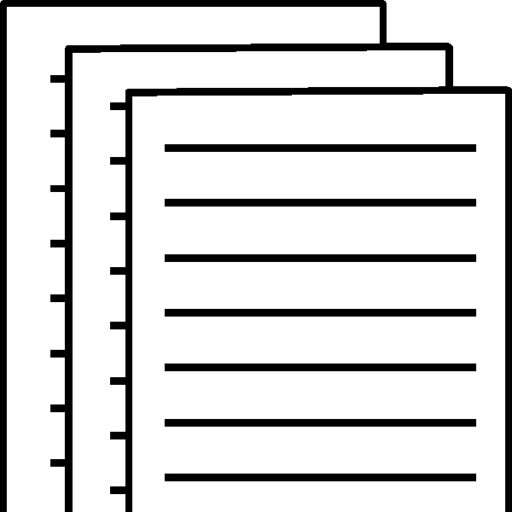## BBA 3310 Unit VI Assignment

Question 1:

 The value of the bond is 814.17

Question 2:

 a. The value of the Enterprise bonds if the interest is paid semiannually is \$ 849.11 b. The value of the Enterprise bonds if the interest is paid annually is \$ 851.61

Question 3:

 The bond’s yield to maturity is 12.41 %

Question 4:

 The bond’s yield to maturity if it matures in 14 years is 8.62 % The bond’s yield to maturity if it matures in 28 years is 8.47 % The bond’s yield to maturity if it matures in 7 years is 8.98 %

Question 5:

 Question Answer a. What is the value of the bond if the markers required yield to maturity on a comparable-risk bond is 8 percent? \$ 893.25 b. What is the value of the bond if the markers required yield to maturity on a comparable-risk bond increases to 11 percent? \$ 663.13 c. What is the value of the bond if the market’s required yield to maturity on a comparable-risk bond decreases to 7 percent? \$ 1000.00 d. The change in the value of a bond caused by changing interest rates is called interest-rate risk. Based on the answer: in parts b and c, a decrease in interest rates (the yield to maturity) will cause the value of a bond to (increase/decrease): Increase By contrast in interest rates will cause the value to (increase/decrease): Decrease Also, based on the answers in part b, if the yield to maturity (current interest rate) equals the coupon interest rate, the bond will sell at (par/face value): Par value exceeds the bond’s coupon rate, the bond will sell at a (discount/premium): Discount and is less than the bond’s coupon rate, the bond will sell at a (discount/premium): Premium e. Assume the bond matures in 5 years instead of 25 years, what is the value of the bond if the yield to maturity on a comparable-risk bond is 8 percent? \$ 960.07 Assume the bond matures in 5 years instead of 25 years, what is the value of the bond if the yield to maturity on a comparable-risk bond is 11 percent? \$ 960.07 f. Assume the bond matures in 5 years instead of 25 years, what is the value of the bond if the yield to maturity on a comparable-risk bond is 7 percent? \$ 1000.00 g. From the findings in part e, we can conclude that a bondholder owning a long-term bond is exposed to (more/less) interest-rate risk than one owning a short-term bond. More

Question 6:

 The firm’s growth rate will be 7.7 %

Question 7:

 a. If your required rate of return is 8.70 percent, the value of the stock for you is: \$ 50.64 b. You (should/should not) make the investment if your expected value of the stock is (greater/less) than the current market price because the stock would be undervalued. Investment should not be made if the intrinsic value does not exceed the market price, thus share is over-valued. Investment should be made if the intrinsic exceeds the market price, thus share is under-valued.

Question 8:

 a. The firm’s growth rate will be: 8.14% b. If the firm decides to increase its retention ratio, what will happen to the value of its common stock? An increase in the retention rate will (increase/decrease) the rate of growth in dividends, which in turn will (increase/decrease) the value of the common stock. Increasing of the retention rate will increase the rate of growth. As a result, value of the common stock is increased. Decreasing of retention rate will increase the rate of growth. As a result, value of the common stock is decreased.

Question 9:

 a. The stock price using the P/E ratio valuation method is: \$ 68.57 b. The stock price using the dividend discount model is: \$ 68.57

Question 10:

 a. The value of the preferred stock is \$61.54 Per share

To view and download a complete answer, scroll down to the bottom to pay